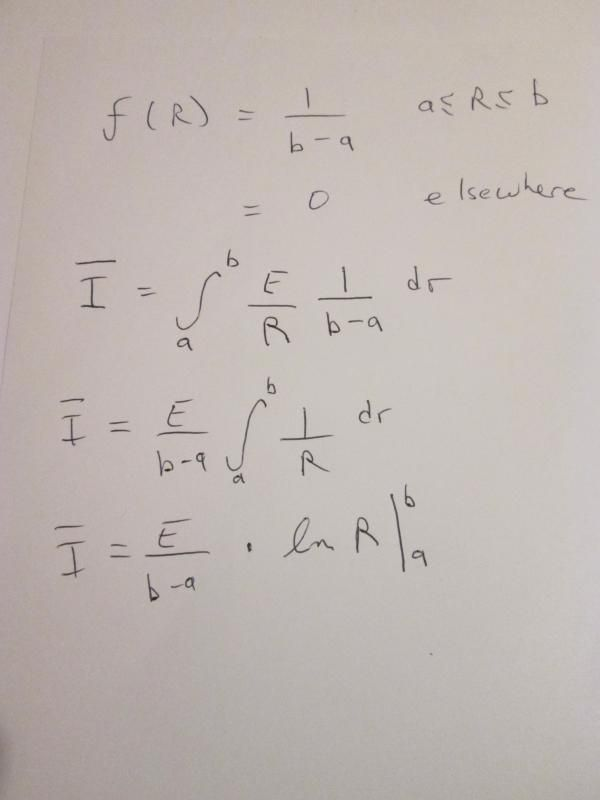# Mean of Current I with Random variable of R

#### Yashodhar.rao

##### New Member
Hi all,
I am new to probability and not so good at it, So please bear with meIf a voltage e is connected across a resistor whose resistance is random variable R, and uniformly distributed between a and b, I needed to find the Mean of current "I" given by "I = e/R"

My question is can an estimate i.e E{I} be given?? as what my notion was that the Estimate can only be given on Random variables and here, I is not given as a random variable.
Secondly, Since is PDF of R is not given how should I consider f(x) of R as?

Yashodhar Rao

#### asterisk

##### New Member#### Yashodhar.rao

##### New Member
Ah!.. thank you asterisk.. I had completely forgotten that "Uniform distribution" was given there!